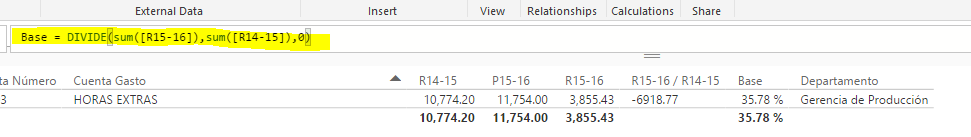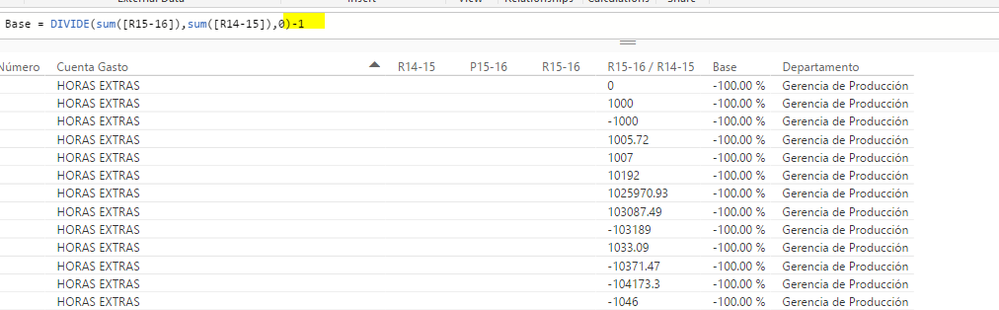cancel
Showing results for
Did you mean:Frequent Visitor

## Excel Formula to PowerBI

Hi, I am new here...

I have this formula in Excel +P12/N12-1, and now I am trying to use it en PowerBI desktop and it doesn't work.

My calculated formula in PowerBI  is> Base = DIVIDE(sum([R15-16]),sum([R14-15]),0) and I need to add the -1

Hope you can help me!

1 ACCEPTED SOLUTIONCommunity Champion

I may be mixing up blank/zero parsing... Try

```Base = IF(
ISBLANK(SUM([R14-15])),
BLANK(),
DIVIDE(
SUM([R15-16]),
SUM([R14-15]),
0
) - 1
)```

Proud to be a Super User!

8 REPLIES 8Community Champion

Have you tried literally just adding the -1 to the formula?

Proud to be a Super User!Frequent Visitor

Sure, but then I lost the group and

See the results:Community Champion

Base = DIVIDE(sum([R15-16]),sum([R14-15]),0) - 1

Proud to be a Super User!Frequent Visitor

When I do it in this way, the group is totally lost and I get my result and the other data from another rows...

See the image:Community Champion

All those rows that show -100% have blank values in R14-15 and R15-16, so DIVIDE returns zero. Zero minus one is negative one. Negative one is negative one hundred percent. So those results are correct for the formula you wanted to replicate. That's what your excel formula would return given the same data. If you'd like those rows to return 0 instead of -1, you could do something like

```Base = IF(
SUM([R14-15]) = 0,
0,
DIVIDE(
SUM([R15-16]),
SUM([R14-15]),
0
) - 1
)```

Proud to be a Super User!Frequent Visitor

The same result that Base = DIVIDE(sum([R15-16]),sum([R14-15]),0) - 1Community Champion

I may be mixing up blank/zero parsing... Try

```Base = IF(
ISBLANK(SUM([R14-15])),
BLANK(),
DIVIDE(
SUM([R15-16]),
SUM([R14-15]),
0
) - 1
)```

Proud to be a Super User!Frequent Visitor

Thank you soo much!!! It worked fine!